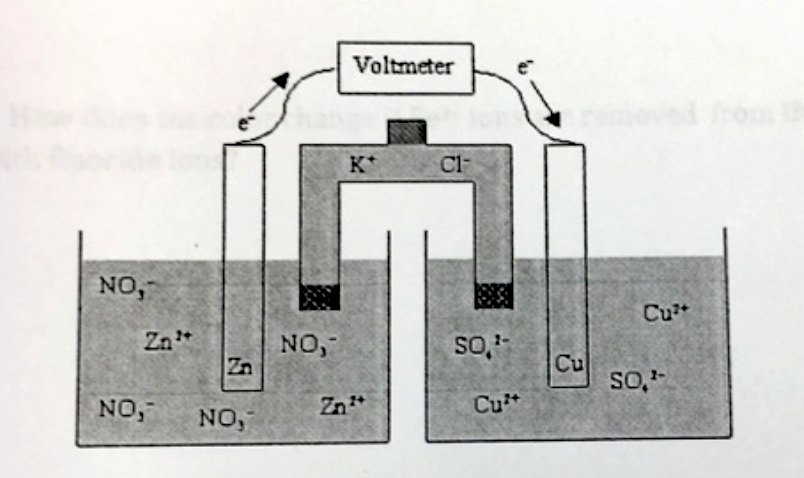# Problem: Which of the following statements is true concerning the voltaic cell shown below? a. The Zn anode mass decreases as the cell discharges.b. The Zn anode mass increases as the cell discharges. c. The Zn cathode mass increases as the cell discharges.d. The Zn cathode mass decreases as the cell discharges. e. The mass of the Zn electrode neither increases nor decreases as the cell discharges.

###### FREE Expert Solution
82% (210 ratings)
###### FREE Expert Solution
82% (210 ratings)###### Problem Details

Which of the following statements is true concerning the voltaic cell shown below?a. The Zn anode mass decreases as the cell discharges.

b. The Zn anode mass increases as the cell discharges.

c. The Zn cathode mass increases as the cell discharges.

d. The Zn cathode mass decreases as the cell discharges.

e. The mass of the Zn electrode neither increases nor decreases as the cell discharges.

What scientific concept do you need to know in order to solve this problem?

Our tutors have indicated that to solve this problem you will need to apply the Galvanic Cell concept. You can view video lessons to learn Galvanic Cell. Or if you need more Galvanic Cell practice, you can also practice Galvanic Cell practice problems.

What is the difficulty of this problem?

Our tutors rated the difficulty ofWhich of the following statements is true concerning the vol...as high difficulty.

How long does this problem take to solve?

Our expert Chemistry tutor, Dasha took 2 minutes and 31 seconds to solve this problem. You can follow their steps in the video explanation above.

What professor is this problem relevant for?

Based on our data, we think this problem is relevant for Professor Madison's class at PITT.